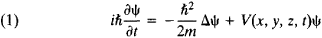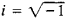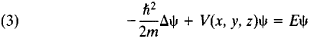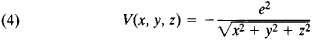# Schrödinger Equation

(redirected from Shrodinger equation)
Also found in: Dictionary, Thesaurus.

## Schrödinger equation

[′shrād·iŋ·ər i‚kwā·zhən]
(quantum mechanics)
A partial differential equation governing the Schrödinger wave function ψ of a system of one or more nonrelativistic particles; h (∂ψ/∂ t) = H ψ, where H is a linear operator, the Hamiltonian, which depends on the dynamics of the system, and h is Planck's constant divided by 2π.

## Schrödinger Equation

the fundamental dynamic equation of nonrelativistic quantum mechanics (seeQUANTUM MECHANICS); named in honor of the Austrian physicist E. Schrödinger, who proposed it in 1926. In quantum mechanics the Schrödinger equation plays the same fundamental role as Newton’s equation of motion plays in classical mechanics and the Maxwell equations play in the classical theory of electromagnetism. The Schrödinger equation describes the time variation of the state of quantum objects, which is characterized by the wave function. If the wave function ψ at the initial moment of time is known, then by solving the Schrödinger equation it is possible to find ψ at any subsequent time t.

For a particle of mass m moving under the action of a force generated by the potential V(x, y, z, t), the Schrödinger equation has the formwhere, h ≅ 1.05 × 10–27 erg·sec is Planck’s constant, and Δ = ∂2/∂x2 + ∂2/∂y2 + ∂2/∂z2 is the Laplace operator (x, y, and z are the coordinates). This equation is called the Schrödinger time-dependent equation.

If the potential V is independent of time, the solutions of the Schrödinger equation may be represented in the form

(2) ψ(x, y, z, t) = e–(i/h)Etψ(x, y, z)

where E is the total energy of the quantum system and ψ (x, y, z) satisfies the stationary Schrödinger equation:For quantum systems whose motion occurs in a bounded region of space, solutions of the Schrödinger equation exist only for certain discrete energy values: E1, E2, . . ., En, . . . ; the members of this series, which is virtually infinite, are numbered by the set of integral quantum numbers n. A wave function ψ(x, y, z) corresponds to every value of En, and a knowledge of the complete set of these functions makes it possible to calculate all measurable characteristics of the quantum system.

In the important particular case of the Coulomb potential(where e is the elementary electric charge), the Schrödinger equation describes the hydrogen atom, and the En are the energies of the stationary states of the atom.

The Schrödinger equation is a mathematical expression of a fundamental property of microparticles—the wave-particle duality—according to which all particles of matter that exist in nature are also endowed with wave properties (this hypothesis was first advanced by L. de Broglie in 1924).

The Schrödinger equation satisfies the correspondence principle and, in the limiting case where the de Broglie wavelengths are much smaller than the dimensions characteristic of the motion under examination, describes particle motion according to the laws of classical mechanics. The transition from the Schrödinger equation to classical trajectories is similar to the transition from wave optics to geometrical optics. The analogy between classical mechanics and geometrical optics, which is the limiting case of wave optics, played an important role in establishing the Schrödinger equation.

From the mathematical standpoint, the Schrödinger equation is a wave equation and is similar in structure to the equation that describes the vibrations of a weighted string. In contrast, however, to the solutions of the equation of the vibration of a string, which give the geometrical shape of the string at a given time, the solutions ψ(x, y, z, t) of the Schrödinger equation do not have direct physical meaning. What has meaning is the square of the wave function, namely, the quantity pn(x, y, z, t) = |ψn(x, y z, t) |2, which is equal to the probability of finding a particle (or system) at a time t in the quantum state n at a point of space having the coordinates x, y, z. This probabilistic interpretation of the wave function is one of the principal postulates of quantum mechanics.

The mathematical formulation of the postulates of quantum mechanics that is based on the Schrödinger equation is called wave mechanics. It is fully equivalent to the matrix mechanics of W. Heisenberg, formulated in 1925.

The Schrödinger equation makes it possible to explain and predict a large number of phenomena of atomic physics and to calculate the experimentally observable fundamental characteristics of atomic systems, such as the energy levels of atoms and the change in atomic spectra under the influence of electric and magnetic fields. By using the Schrödinger equation, it has also been possible to understand and describe quantitatively a broad range of phenomena in nuclear physics, such as the regularities underlying alpha decay, the gamma radiation of nuclei, and the scattering of neutrons by nuclei.

### REFERENCE

Schrödinger, E. Novye puti v fizike: Stat’i i rechi. Moscow, 1971.

L. I. PONOMAREV

Site: Follow: Share:
Open / Close